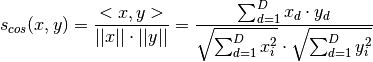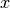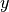Shortcuts

# Cosine Similarity¶

## Functional Interface¶

torchmetrics.functional.pairwise_cosine_similarity(x, y=None, reduction=None, zero_diagonal=None)[source]

Calculates pairwise cosine similarity:If bothandare passed in, the calculation will be performed pairwise between the rows ofand. If onlyis passed in, the calculation will be performed between the rows of.

Parameters
• x (Tensor) – Tensor with shape [N, d]

• y (Optional[Tensor]) – Tensor with shape [M, d], optional

• reduction (Optional[Literal[‘mean’, ‘sum’, ‘none’, None]]) – reduction to apply along the last dimension. Choose between ‘mean’, ‘sum’ (applied along column dimension) or ‘none’, None for no reduction

• zero_diagonal (Optional[bool]) – if the diagonal of the distance matrix should be set to 0. If onlyis given this defaults to True else ifis also given it defaults to False

Return type

Tensor

Returns

A [N,N] matrix of distances if only x is given, else a [N,M] matrix

Example

>>> import torch
>>> from torchmetrics.functional import pairwise_cosine_similarity
>>> x = torch.tensor([[2, 3], [3, 5], [5, 8]], dtype=torch.float32)
>>> y = torch.tensor([[1, 0], [2, 1]], dtype=torch.float32)
>>> pairwise_cosine_similarity(x, y)
tensor([[0.5547, 0.8682],
[0.5145, 0.8437],
[0.5300, 0.8533]])
>>> pairwise_cosine_similarity(x)
tensor([[0.0000, 0.9989, 0.9996],
[0.9989, 0.0000, 0.9998],
[0.9996, 0.9998, 0.0000]])


© Copyright Copyright (c) 2020-2023, Lightning-AI et al... Revision 825d17f3.

Built with Sphinx using a theme provided by Read the Docs.
Versions
latest
stable
v0.11.3
v0.11.2
v0.11.1
v0.11.0
v0.10.3
v0.10.2
v0.10.1
v0.10.0
v0.9.3
v0.9.2
v0.9.1
v0.9.0
v0.8.2
v0.8.1
v0.8.0
v0.7.3
v0.7.2
v0.7.1
v0.7.0
v0.6.2
v0.6.1
v0.6.0
v0.5.1
v0.5.0
v0.4.1
v0.4.0
v0.3.2
v0.3.1
v0.3.0
v0.2.0
v0.1.0